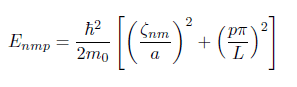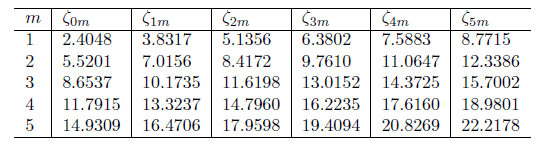# Bessel Function Zeros - To find Energy Levels

LiorSh
[Mentors' note: Moved from the technical forums, so no template]

Hi,

I have to find energy levels of an electron in a cylindrical shape. I know how to derive the formula below:However, I'm not sure which zero value and what intger p I need to use in order to find the lowest energy.
If these are the bessel fucntion zeros:Lets say, I want to find the lowest energy - Do I need to use the value of 2.4048 and p =1 ? What about the second lowest? would I use 5.5201 and p=2?
Thanks!

Best,
Lior

#### Attachments

Last edited by a moderator:

## Answers and Replies

Homework Helper
Gold Member
[Mentors' note: Moved from the technical forums, so no template]

Hi,

I have to find energy levels of an electron in a cylindrical shape. I know how to derive the formula below: View attachment 228070
However, I'm not sure which zero value and what intger p I need to use in order to find the lowest energy.
If these are the bessel fucntion zeros:
View attachment 228071

Lets say, I want to find the lowest energy - Do I need to use the value of 2.4048 and p =1 ?

What values can p take? If the smallest value is 1 then yes, your choice gives the lowest energy.

What about the second lowest? would I use 5.5201 and p=2?
Thanks!

Best,
Lior
Why do you consider 5.5201 instead of 3.8317??
For the second lowest, the answer depends on the ratio a/L. Without that value, we cannot tell for sure.

LiorSh
a is 3nm, and L is 5nm. Also I thought that only the first column is for a symmtric cylinder, and the second colum is for a general soultion only. Isn't that the case? Thank you!
What values can p take? If the smallest value is 1 then yes, your choice gives the lowest energy.

Why do you consider 5.5201 instead of 3.8317??
For the second lowest, the answer depends on the ratio a/L. Without that value, we cannot tell for sure.

Homework Helper
Gold Member
a is 3nm, and L is 5nm. Also I thought that only the first column is for a symmtric cylinder, and the second colum is for a general soultion only. Isn't that the case? Thank you!
The potential is symmetrical, but the solutions do not have to be (for example a state with angular momentum in the hydrogen atom is not spherically symmetric even though the Coulomb potential is spherically symmetric). However, to answer properly we need to know what the table means, exactly, by "zeroes". Are these the values that give a zero of the wave function at the surface of the cylinder (I would think so)? In that case, all values are possible.

Given the values of "a" and "L", look at what happens when you increase p but keep n,m as they were for the ground state. Then see what happens when you keep p but increase n,m to get the next possible value. One of these two choices will give a smaller value than the other and that will be the second lowest energy state.

LiorSh
The potential is symmetrical, but the solutions do not have to be (for example a state with angular momentum in the hydrogen atom is not spherically symmetric even though the Coulomb potential is spherically symmetric). However, to answer properly we need to know what the table means, exactly, by "zeroes". Are these the values that give a zero of the wave function at the surface of the cylinder (I would think so)? In that case, all values are possible.

Given the values of "a" and "L", look at what happens when you increase p but keep n,m as they were for the ground state. Then see what happens when you keep p but increase n,m to get the next possible value. One of these two choices will give a smaller value than the other and that will be the second lowest energy state.
So these are my results:
for p = 1, and zero value = 2.4048, I for sure get the lowest energy, and the logic make sense. I took the lowest possible values of p and the zeros.
then for the second lowest, p=2, and zero=2.4048, and for the thrid I got p=1, and zero = 5.5201.

Doest it make sense?

Homework Helper
Gold Member
So these are my results:
for p = 1, and zero value = 2.4048, I for sure get the lowest energy, and the logic make sense. I took the lowest possible values of p and the zeros.
then for the second lowest, p=2, and zero=2.4048, and for the thrid I got p=1, and zero = 5.5201.

Doest it make sense?
Again, all the zeros are allowed, you don't have to force n=0. That will change your answer.

LiorSh
I'm not sure I completely understand. Is there some logic to which pairs I should pick? I mean, there is an infinite number of combinations.

Homework Helper
Gold Member
I'm not sure I completely understand. Is there some logic to which pairs I should pick? I mean, there is an infinite number of combinations.
you try a few values and it will be clear which one is the smallest. Clearly for the ground state, p=1 and n,m=0,1 gives the smallest value.

For the second lowest, just check what happens when you increase each of the quantum numbers separately, so try p=2,(n,m)=0,1 and then p=1,(n,m)=1,1 and then p=1, (n,m)=0,2. These are the only three sensible things to try for the second lowest.

For the next one, you look at all the possibilities of increasing two of the quantum numbers from the ground state. There are more possibilities, for example try p=3, (n,m)=0,1 then try p=2, (n,m)=(1,1) then p=2, (n,m)=2,1, etc (for a given p, you can see directly from the table what you should pick for n,m, no need to do a calculation).etc There are many cases to consider but not an infinity!

LiorSh
Yes, that's what I thought. It makes sense now :) Thank you so much!